Home > CCA2 > Chapter 11 > Lesson 11.1.1 > Problem11-9

11-9.
1. Consider the function f(x) = ex. Homework Help ✎

1. What is its inverse function, f −1(x)?

2. Investigate f(x) and f −1(x).

3. For what integer value of n is the graph of f −1(x) between the graphs of y = lognx and y = logn+1x?

4. For what values of x is the graph of f −1(x) above each of the graphs in your answer to part (c)? For what x-values is it below?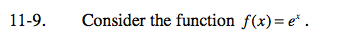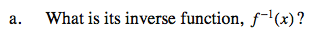If the function is y = ex the the inverse function is x = ey. Now solve for y.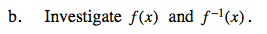Graph each function. State each function's domain, range, asymptote(s), and intercept(s).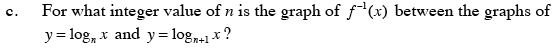ln x = logex

What is the value of e?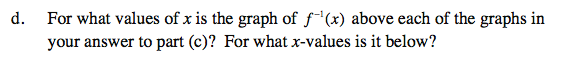When does ln(x) = log2(x) = log3(x)?